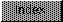```

NAME
SPSincos - obtain the sine and cosine of a number

SYNOPSIS
fnum3 = SPSincos(pfnum2, fnum1);
d1.l,   d0.l
float *pfnum2;
float fnum1;
float fnum3;

FUNCTION
Accepts a floating point number (fnum1) representing
an angle in radians and a pointer to another floating
point number (pfnum2). It computes the cosine and places it in
*pfnum2. It computes the sine and returns it as a result.

INPUTS
fnum1 - Motorola fast floating point number
pfnum2 - pointer to Motorola fast floating point number

RESULT
*pfnum2 - Motorola fast floating point number (cosine)
fnum3 - Motorola fast floating point number (sine)

BUGS
None